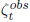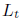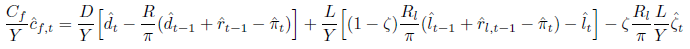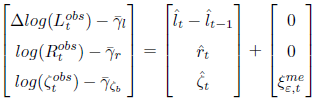# Specifying Measurement Equation

Dear Dynare Team,

I have read through “A Guide to Specifying Observation Equations for the Estimation of DSGE Models” and other relevant posts in this forum regarding this topic. I just want to confirm that what I am doing is correct. I have the following observable variables in my model: demeaned log differences of real GDP (Y_(obs,t)) and demeaned log values of gross inflation rate (\Pi_(obs,t)) and demeaned log values of gross quarterly nominal interest rate (R_(obs,t)). \Pi_(obs,t) = 1 + \pi_(obs,t)/100 , where \pi_(obs,t) is quarterly inflation rate measured by % change in quarterly CPI. R_(obs,t) = 1 + r_(obs,t)/400, where r_(obs,t) is annual interest rate. I have specified the measurement equation as follows:

where \gamma_i represents the corresponding sample mean of variable i and y_hat, pi_hat and r_hat are the corresponding log-linearised variables appearing in the model. Can someone confirm whether this is correct based on how I have constructed the data.

Regards,

Ernest

That looks mostly correct. But you need to check the scaling by 100. It seems you multiplied everything by 100 except the resulting interest rate. Also, you need to demean after scaling.

Dear Prof Pfeifer,

Thanks for your prompt response. It was a mistake on my part, I forgot to scale the resulting interest rate by 100. Here is the correct measurement equations:

I did demean the variables after scaling. That is,. I did the same with the other variables.

Ok. Then that should be correct

Thanks very much for your assistance Prof Pfeifer.

Dear Prof Pfeifer,

Can you please confirm whether I have correctly mapped the empirical data (actual times series) with the corresponding model variable, especially for the ratio of non-performing loans (), which is expressed as a net rate. That is,is always positive.
To give some details, I have the bank’s budget constraint as follows;whereis loan amount,is gross lending rate andis the ratio of non-performing loans. The above is log-linearized as follows:Then, I specified the measurement equation as follows:whereis the temporal difference operator andis the sample mean of the respective transformed variables.is measurement error.

Regards,
Ernest

The way you map demeaned growth rates in the data to mean zero growth rates in the model is correct. The only potential issue is the frequency of the lending rate, i.e whether is annualized in the data and quarterly in the model

Thank you very much for the response Prof Pfeifer,

Interest rate is transformed as follows: R_(obs,t) = 1 + r_(obs,t)/400, where r_(obs,t) is annual net interest rate.

Regards,

Ernest

That sounds correct.

Thank you very much for your assistance Prof Pfeifer.

Regards,

Ernest

Good morning Enerst,
i would like to know if it is the variable PI_obs or the variable CPI in the measurement equation
best,

Dear Kacou225,

In equation C.1 above (see previous post), I have demeaned log values of gross inflation rate (Pi_(obs,t)). Pi_(obs,t) ≡ 100log(CPI_(obs,t)) is gross quarterly inflation rate measured by a % change in quarterly CPI.

In short, I have a variable PI_obs (as explained above) in the measurement equation C.1.

Hope this helps.

1 Like